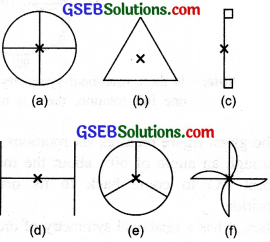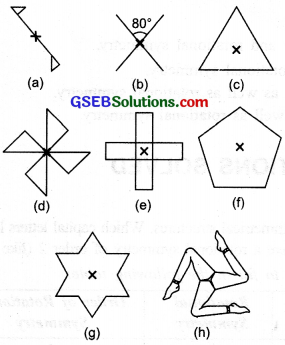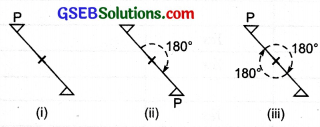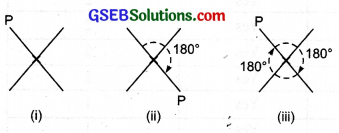# GSEB Solutions Class 7 Maths Chapter 14 Symmetry Ex 14.2

Gujarat Board GSEB Textbook Solutions Class 7 Maths Chapter 14 Symmetry Ex 14.2 Textbook Questions and Answers.

## Gujarat Board Textbook Solutions Class 7 Maths Chapter 14 Symmetry Ex 14.2Question 1.
Which of the following figures have rotational symmetry of order more than 1:Solution:
The figures (a), (b), (d), (e) and (f) have rotational symmetry of order more than 1.Question 2.
Give the order of rotational symmetry for each figure:Solution:
(a)Let us mark a point P as shown in the figure (i). It requires two rotations, each through 180° about the point (X) to come back to its original position.
∴ It has a rotational symmetry of order 2.

(b)Mark a point P as shown is figure (i). It requires two rotations, each through an angle of 180°, about the marked point (X) to come back to its original position.
Thus, it has a rotational symmetry of order 2.

(c)Mark a point P as shown in figure (i) It requires three rotations, each through an angle of 120°, about the marked point (X) to come back to its original position.
Thus, it has a rotational symmetry of order 2.

(d)Mark a point P as shown in Fig. (i). It requires four rotations, each through an angle of 90°, about the marked point (X) to come back to its original position.
Thus, it has a rotational symmetry of order 4.

Similarly,

(e) The figure requires four rotations each of 90°, about the marked point (X) to come back to its original position.
∴ It has a rotational symmetry of order 4.

(f) The figure is a regular pentagon. It requires five rotations, each through an angle of 72°, about the marked point to come back to its original position.
∴ It has rotational symmetry of order 5.

(g) The given figure requires six rotations, each through an angle of 60°, about the marked point (X) to come back to its original position.
Thus, it has a rotational symmetry of order 6.

(h) The given figure requires three rotations, each through an angle of 120°, about the marked point (X), to come back to its original position.
∴ Its has a rotational symmetry of order 3.